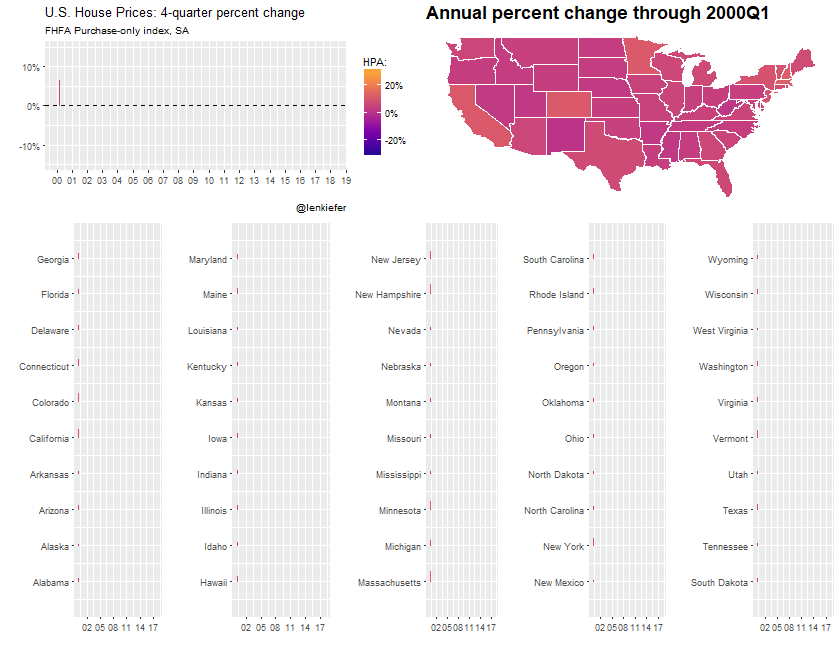# Plotting house price and income trends

## In this post we will create some plots of house prices and incomes for the United States and individual states. We will also try out the bea.R package to get data from the U.S. Bureau of Economic Analysis..

In this post we will create some plots of house prices and incomes for the United States and individual states. We will also try out the bea.R package to get data from the U.S. Bureau of Economic Analysis.

We’ll end up with something like this: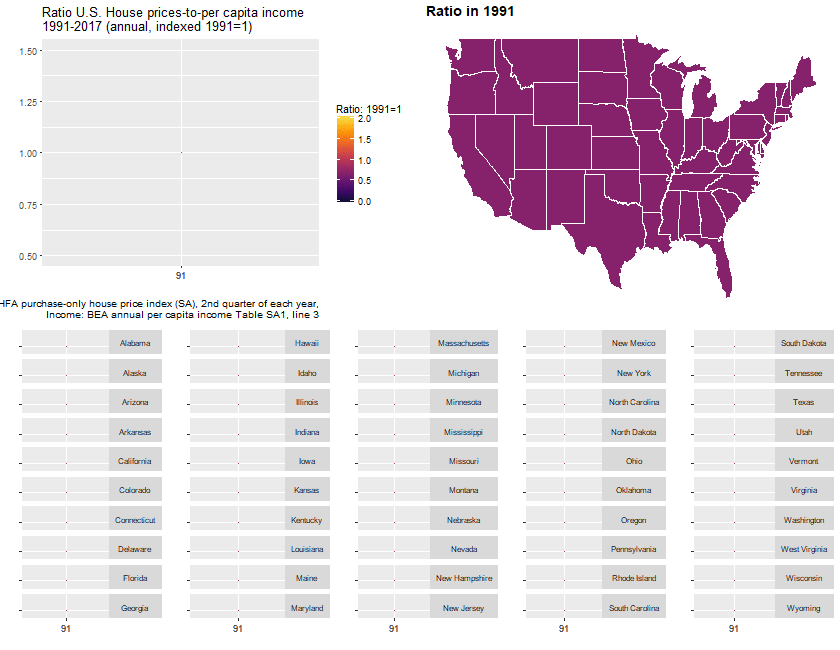Per usual we’ll do it with R and I’ll include code so you can follow along.

# Data

We’re going to use two sources of data. First, we’ll get the FHFA house price index and then we’ll get per capita income estimates from the United States Bureau of Economic Analysis (BEA).

We could get most of these data via the Saint Louis Federal Reserve’s FRED. While FRED is a great resource, as much as possible I like to go directly to the source for the data. This also gives us a chance to work with the bea.R package, available on CRAN.

Let’s get to wrestling the data.

## House Price Index

The house price index data we want is available via a flat text file on the FHFA webpage (large .csv file). We can use the data.table function data.table::fread() to read the data in. Then we’ll filter the file down to the quarterly purchase-only house price index for states and the United States.

# Load Libraries ----
library(data.table)
library(tidyverse)
library(viridis)
library(bea.R)
library(tigris) # for maps
library(cowplot)
library(animation) #for animation
library(ggthemes)

# This gets all hpi data
# Take a look
knitr::kable(head(df.hpi))
hpi_type hpi_flavor frequency level place_name place_id yr period index_nsa index_sa
traditional purchase-only monthly USA or Census Division East North Central Division DV_ENC 1991 1 100.00 100.00
traditional purchase-only monthly USA or Census Division East North Central Division DV_ENC 1991 2 100.99 101.09
traditional purchase-only monthly USA or Census Division East North Central Division DV_ENC 1991 3 101.35 101.02
traditional purchase-only monthly USA or Census Division East North Central Division DV_ENC 1991 4 101.76 101.07
traditional purchase-only monthly USA or Census Division East North Central Division DV_ENC 1991 5 102.38 101.45
traditional purchase-only monthly USA or Census Division East North Central Division DV_ENC 1991 6 102.80 101.56

There are several files detailing information about the house price index. Additional details can be found here.

We want to grab the traditional type, purchase-only flavor, quarterly frequency. We’ll set up two data.frames that are subsets of the large data. One for the United States and one for the states.

# get quarterly purchase-only index for the United States
df.usa <-df.hpi[frequency=="quarterly" & hpi_flavor=="purchase-only" & place_name==  "United States",][, hpa:=index_sa/shift(index_sa,4)-1][,date:=as.Date(ISOdate(yr,period*3,1))]

# get quarterly purchase-only state indices
df.st <- df.hpi[frequency=="quarterly" & hpi_flavor=="purchase-only" & level=="State",]
df.st <- df.st[,date:=as.Date(ISOdate(yr,period*3,1))]

# Drop D.C.
df.st <- df.st[place_name !="District of Columbia",]
df.st <- df.st[, place_namef:=factor(place_name)]

## BEA data

Let’s set aside the house price data for now and go get income data from the BEA. We’ll use the bea.R package. In order for this to work for you, make sure you get an API key as detailed in the bea.R documentation. Once you have a key, you’ll then need to figure out what table you need and how to use the API. This example could help.

For example, per capita income estimates can be found in table SQ1.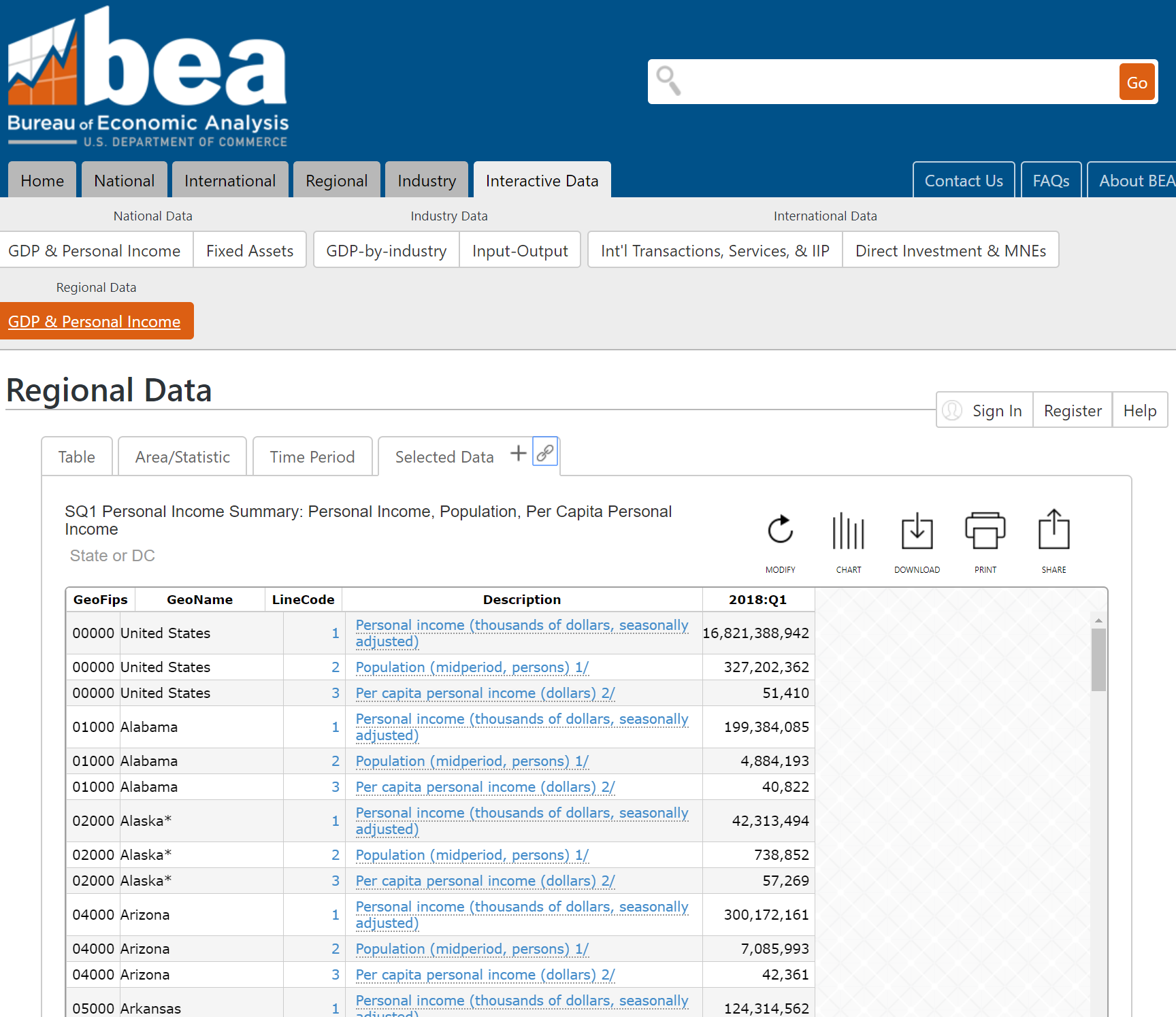beaKey  <- 'YOUR-API-KEY' #need a key from BEA
# get annual data for US
beaSpecs <- list(
'UserID' = beaKey ,
'Method' = 'GetData',
'datasetname' = 'RegionalIncome',
'GeoFIPS' = '00000',
'TableName' = 'SA1',
'Frequency' = 'A',
'Year' = 'ALL',   # All available years
'ResultFormat' = 'json',
'LineCode' = '3'  # variable, line 3 corresponds to per capita income
)

us.pcA <- beaGet(beaSpecs, asWide=FALSE)   #query BEA, use a wide format
us.pcA <-  mutate(us.pcA, yr=substr(TimePeriod,1,4), qtr=2) %>% mutate(date=as.Date(ISOdate(as.numeric(yr),as.numeric(qtr)*3,1)))

# get quarterly data for US
beaSpecs <- list(
'UserID' = beaKey ,
'Method' = 'GetData',
'datasetname' = 'RegionalIncome',
'GeoFIPS' = '00000', #US
'TableName' = 'SQ1',
'Frequency' = 'Q', # quarterly frequency
'Year' = 'ALL',
'ResultFormat' = 'json',
'LineCode' = '3'  # variable, line 3 corresponds to per capita income
)
us.pcQ <- beaGet(beaSpecs, asWide=FALSE) %>% filter(!is.na(DataValue))
us.pcQ <- mutate(us.pcQ, yr=substr(TimePeriod,1,4), qtr=substr(TimePeriod,6,6)) %>% mutate(date=as.Date(ISOdate(as.numeric(yr),as.numeric(qtr)*3,1)))

knitr::kable(tail(us.pcQ))  # prints header of table
Code GeoFips GeoName TimePeriod CL_UNIT UNIT_MULT DataValue NoteRef yr qtr date
28 SQ1-3 00000 United States 2016Q4 dollars 0 49350 NA 2016 4 2016-12-01
29 SQ1-3 00000 United States 2017Q1 dollars 0 49962 NA 2017 1 2017-03-01
30 SQ1-3 00000 United States 2017Q2 dollars 0 50172 NA 2017 2 2017-06-01
31 SQ1-3 00000 United States 2017Q3 dollars 0 50463 NA 2017 3 2017-09-01
32 SQ1-3 00000 United States 2017Q4 dollars 0 50951 NA 2017 4 2017-12-01
33 SQ1-3 00000 United States 2018Q1 dollars 0 51410 NA 2018 1 2018-03-01

To get the states, we need a list of state FIPS codes. I happen to have a text file here that has states FIPS codes and regions. Unfortunately this file only has 2 digit FIPS codes formatted as numbers. We need 5 digit numbers (padded left with a leading zero if less than 10 and 3 trailing zeros).

region <- fread("http://lenkiefer.com/img/charts_feb_20_2017/region.txt")
knitr::kable(head(region))
fips statecode statename division region
9 CT Connecticut New England Division Northeast Region
23 ME Maine New England Division Northeast Region
25 MA Massachusetts New England Division Northeast Region
33 NH New Hampshire New England Division Northeast Region
44 RI Rhode Island New England Division Northeast Region
50 VT Vermont New England Division Northeast Region

Now we can create a list and feed it to the beaSpecs list we created.

st.pcQ$GeoName<- gsub("[*].*$","",st.pcQ$GeoName) And now we are ready to join the data. Using a left assignment I might add. # merge to df.usa q=2 for US data dt.us <- left_join(filter(df.usa,period==2), select(us.pcA ,date,DataValue), by="date") %>% mutate(hpi=index_sa/index_sa[yr==1991], inc=DataValue/DataValue[yr==1991]) %>% ungroup() %>% data.table() # for states join hpi (for Q2) with bea annual income df.combined <- left_join(df.st %>% filter(period==2), st.pcA %>% select(Code,GeoName,GeoFips,CL_UNIT,date,DataValue), by=c("place_name"="GeoName","date"="date")) %>% data.table() df.combined <- left_join(df.combined, region ,by=c("place_id"="statecode")) # compute values relative to 1991 Q4: df.combined %>% group_by(place_id) %>% mutate(hpi=index_sa/index_sa[yr==1991], inc=DataValue/DataValue[yr==1991]) %>% ungroup() %>% data.table() -> dt # Charts As I was making this post, I went down a very dark and twisted path. I eventually ended up trying to replicate ggplot2 facets, using ggplot2! But we can skip most of that business and just use standard facets. You can see the results of that experiment on Twitter: Let’s begin by comparing annual house prices and per capita income for the United States. It will be useful to construct a function. # list of dates dlist2 <- unique(dt.us$date)

gf2.us <- function(i=27){
ggplot(data=dt.us[date>=dlist2 & date<=dlist2[i], ],
aes(x=date,y=hpi,group=place_name))+
geom_line(aes(color="House Prices"),size=1.05)+
geom_line(aes(color="Per capita income", y=inc),linetype=2,size=1.025)+
theme(strip.text.y = element_text(angle = 0, size=8))+
scale_color_manual(name="indicates: ",values=c("#d73027","#4575b4"))+
labs(y="",x="", title="U.S. House prices and per capita income: 1991-2017 (annual, indexed 1991=1)",
caption="@lenkiefer Sources: House prices: FHFA purchase-only house price index (SA), 2nd quarter of each year,\nIncome: BEA annual per capita income Table SA1, line 3")+
theme(legend.position="top")+
scale_x_date(limits=c(min(dt.us$date), max(dt.us$date)),date_breaks="1 year", date_labels="%y")+
scale_y_continuous(breaks=seq(0,2.5,0.5), limits=c(0.5,2.6))

}

gf2.us()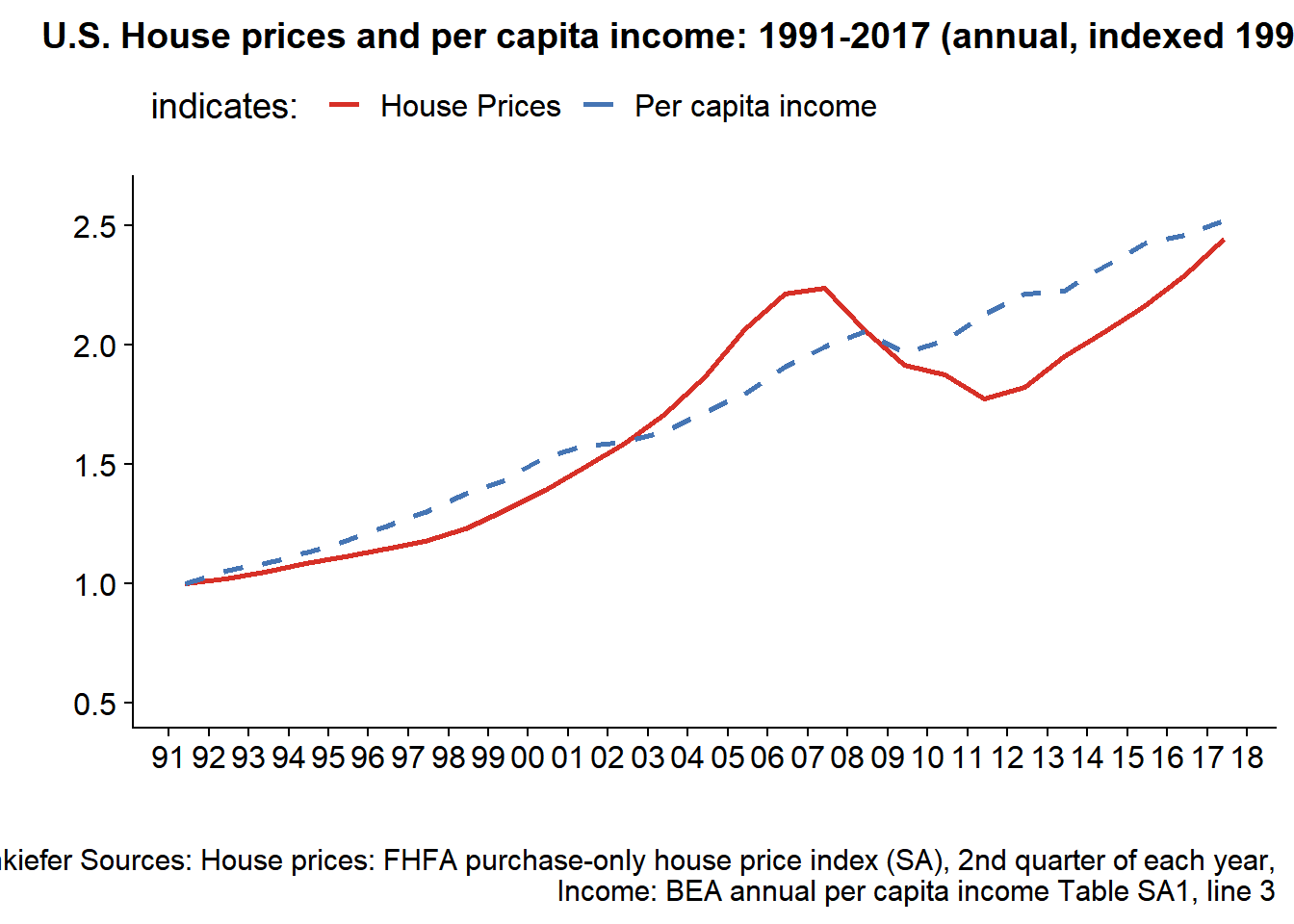Next, we can construct the ratio of these two index values to see how much house prices have moved relative to incomes.

gf.us <- function(i=27){
ggplot(data=dt.us[date>=dlist2 & date<=dlist2[i], ],
aes(x=date,y=hpi/inc,group=place_name))+geom_line()+
theme_gray()+
theme(strip.text.y = element_text(angle = 0, size=8))+
geom_ridgeline_gradient(aes(fill=hpi/inc, height=hpi/inc-1,y=1), color="black", min_height= -1)   +
labs(y="",x="", title="Ratio U.S. House prices-to-per capita income\n1991-2017 (annual, indexed 1991=1)",
caption="@lenkiefer Sources: House prices: FHFA purchase-only house price index (SA), 2nd quarter of each year,\nIncome: BEA annual per capita income Table SA1, line 3")+
scale_fill_viridis(option="B", name="Ratio: 1991=1 ",end=0.9,begin=0.1, limits=c(0,2))  +
theme(legend.position="top")+
scale_x_date(limits=c(dlist2, dlist2[i]),breaks=c(dlist2, dlist2[i]), date_labels="%y")+
scale_y_continuous(breaks=seq(0,2.5,0.25), limits=c(0.5,1.5))

}
gf.us()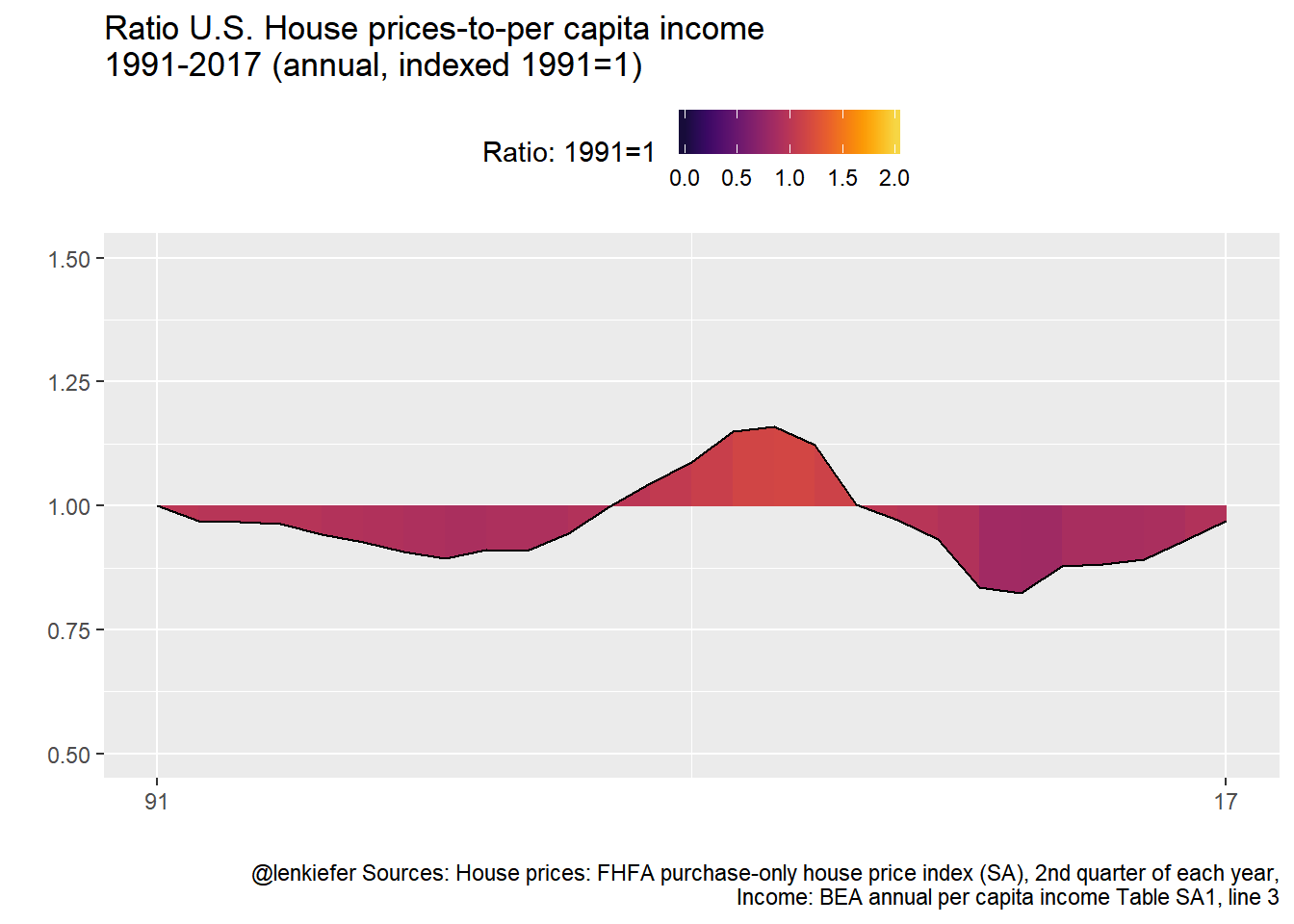It might be instructive to make a map. We’ll use the tigris package to construct a state map for the United States (excluding Alaska and Hawaii).

us_geo2 <- states(cb=TRUE)
us_geo48 <- us_geo2[! us_geo2@data$STUSPS %in% c("AK","PR","VI","HI","GU","MP","AS"), ] us_geo48@data$id <- rownames(us_geo48@data)
us_geo48f <- fortify(us_geo48)
us_geo48f <- left_join(us_geo48f,us_geo48@data, by="id")

Then we can create a map.

gmap.us <- function(i=27){
df.map <- left_join(us_geo48f,dt[date==dlist2[i],], by=c("NAME"="place_name"))
g.map <-
ggplot(data=df.map,
aes(x=long,y=lat, map_id=id, group=group,
fill=hpi/inc))+
geom_polygon(color="white")+theme_map()+
scale_fill_viridis(option="B", name="Ratio: 1991=1 ",  label=scales::percent, end=0.9,begin=0.1, limits=c(.5,2))+
labs(title=paste0("Ratio in ",as.character(dlist2[i],"%Y")))+
theme(legend.position="none",
plot.caption=element_text(hjust=1),
plot.title=element_text(hjust=0,face="bold",size=14))
g.map
}
gmap.us()+
theme(legend.position="top")+
labs(title="House price-to-per capita income ratio in 2017 (1991=1)",caption="@lenkiefer Sources: House prices: FHFA purchase-only house price index (SA), 2nd quarter of each year,\nIncome: BEA annual per capita income Table SA1, line 3")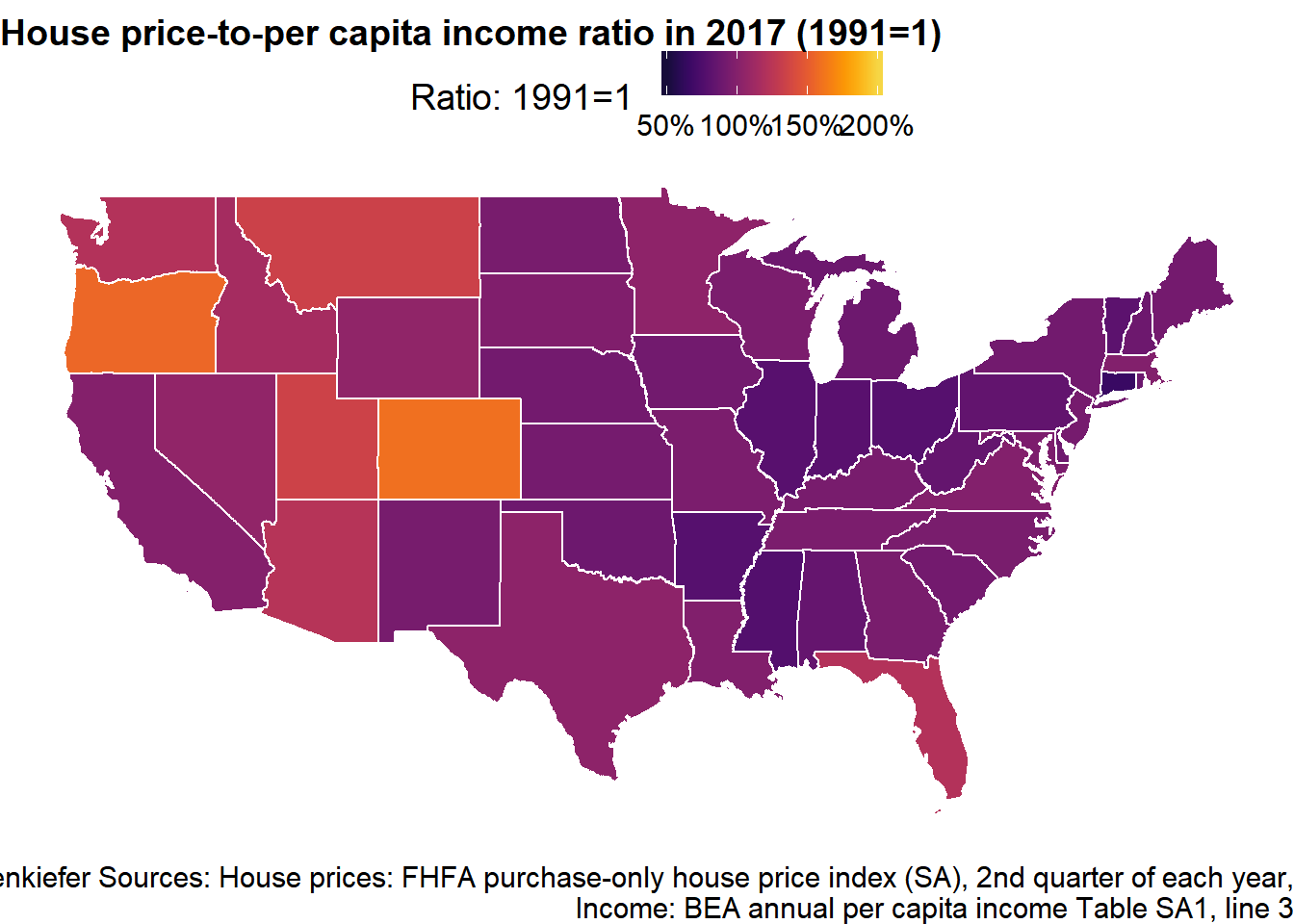Now we can combine our timeline for the United States and map with a set of little timelines for the states.

# create a factor
dt <- dt[, place_namef:=factor(place_name)]
# reverse order of factor:
df.st$place_namef<- factor(df.st$place_namef, levels=rev(levels(df.st$place_namef))) gf <- function(i,in.start,in.end){ ggplot(data=dt[ place_namef %in% levels(dt$place_namef)[in.start:in.end] &  date>=dlist2 & date<=dlist2[i], ],
aes(x=date,y=hpi/inc,group=place_name))+geom_line()+facet_grid(place_name~.)+
theme(strip.text.y = element_text(angle = 0, size=8))+
geom_ridgeline_gradient(aes(fill=hpi/inc, height=hpi/inc-1,y=1), color="black", min_height= -1)   +
labs(y="",x="")+
scale_fill_viridis(option="B", name="Ratio: 1991=1 ",  label=scales::percent,end=0.9,begin=0.1, limits=c(0,2))  +
theme(legend.position="none", axis.text.y=element_blank())+
scale_x_date(limits=c(dlist2[start], dlist2[i]),breaks=c(dlist2[start], dlist2[i]), date_labels="%y")+
scale_y_continuous(breaks=1, limits=c(0.5,2))
}

myf6 <- function(i=27){
g.spark <- cowplot::plot_grid(gf(i,41,50),gf(i,31,40),gf(i,21,30),gf(i,11,20),gf(i,1,10),ncol=5)
cowplot::plot_grid(cowplot::plot_grid(gf.us(i),gmap.us(i),ncol=2),g.spark,ncol=1)
}
myf6()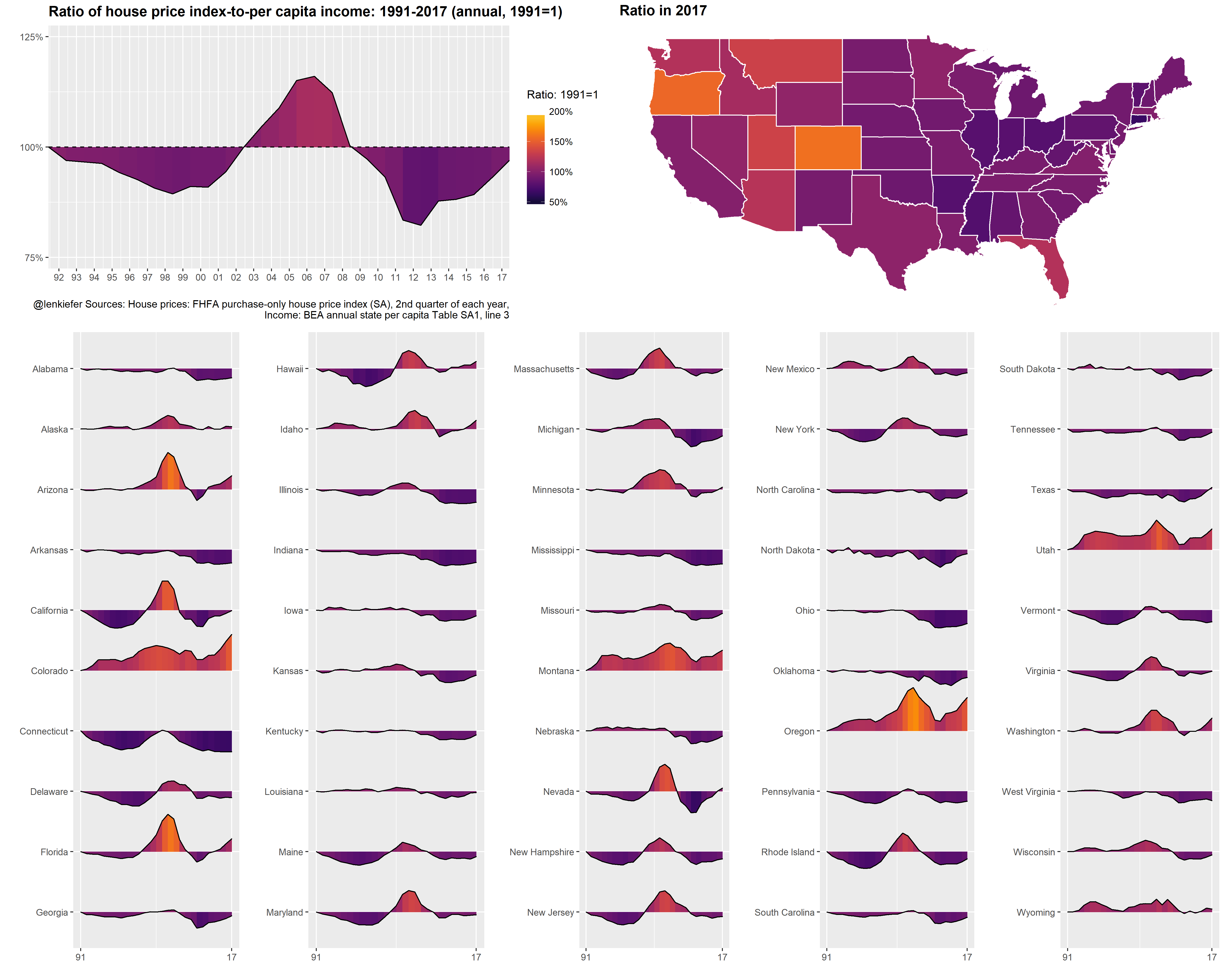And then we can animate it. See here for many posts on animating charts.

saveGIF({for (i in 1:length(dlist2)){

g<-myf6(i)
print(g)
print(paste(i,"out of",length(dlist2)))
ani.pause()
}
for (ii in 1:10){
print(g)
ani.pause()
print(ii)
}

}, movie.name = "spark6.gif", ani.width=840, ani.height=650)You could modify the code above to keep the axis fixed: